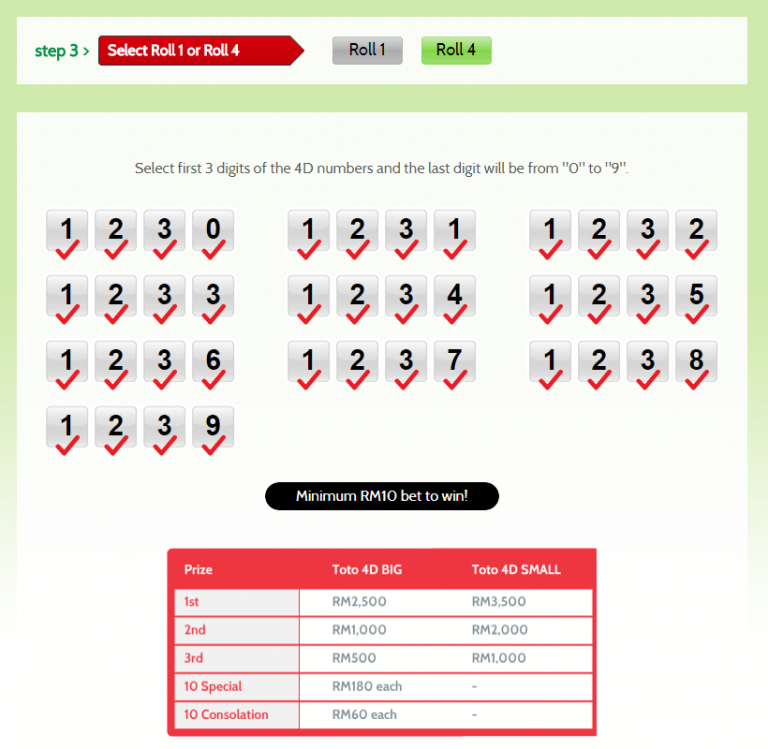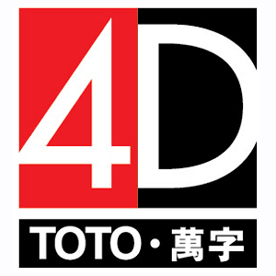Sports TOTO 4D

3 easy steps to winning chances!

1. Standard Game
2. i-Perm / i-Permutation
3. Roll

#### Standard Game Play

A. 4D Standard + BIG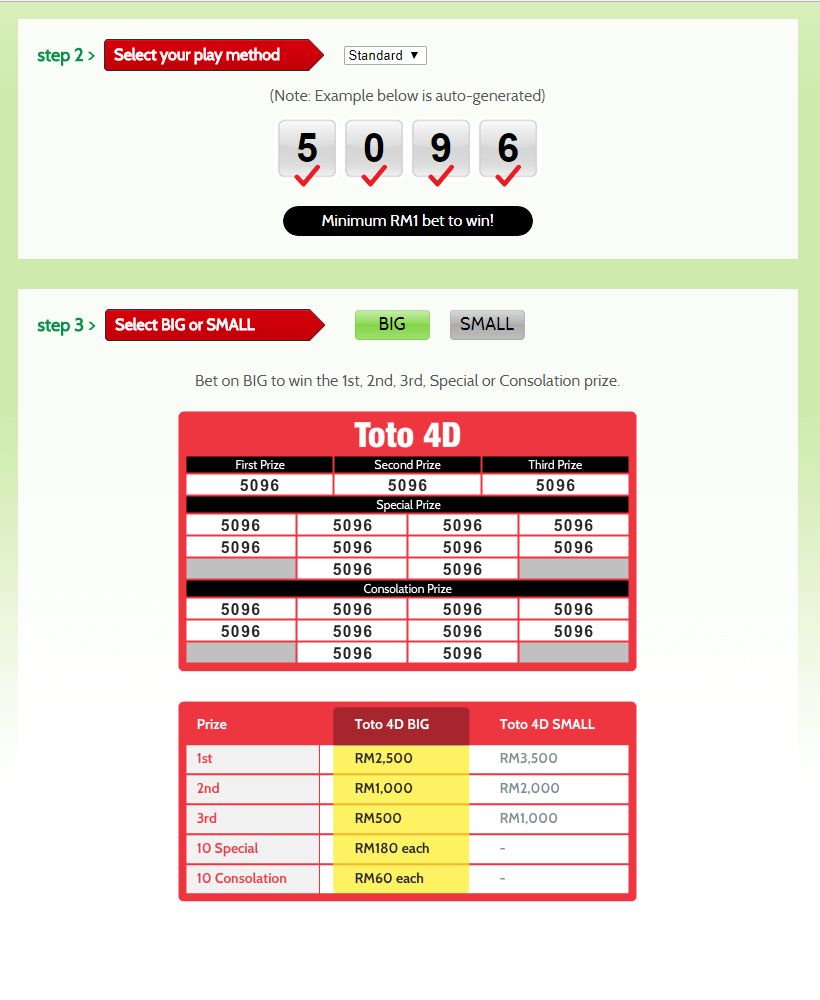B. 4D Standard + SMALL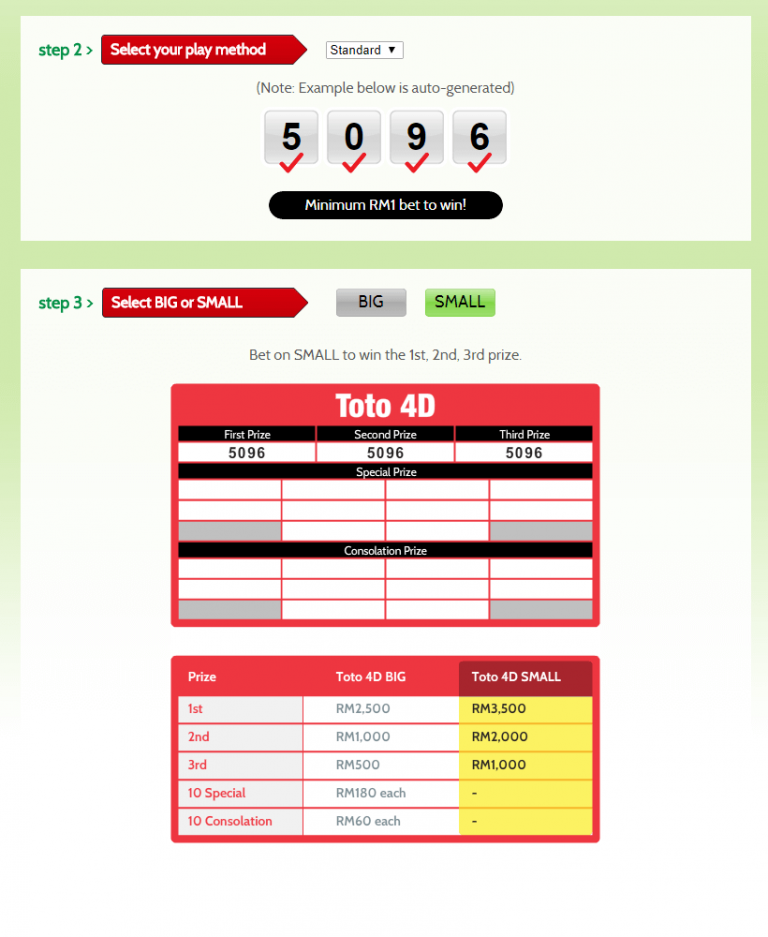4D i-Perm / i-Permutation

i-Perm or Insurance Permutation gives you more chances to win with smaller bets. Each set of permutation costs RM1.00.

Below is an example of a 4-Permutation number which costs RM1.00.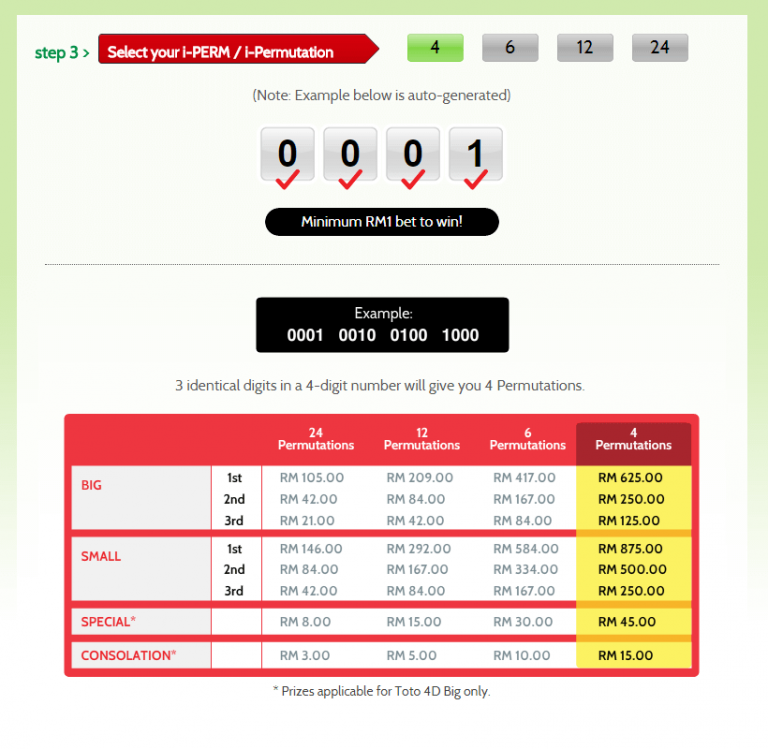Below is an example of a 6-Permutation number which costs RM1.00.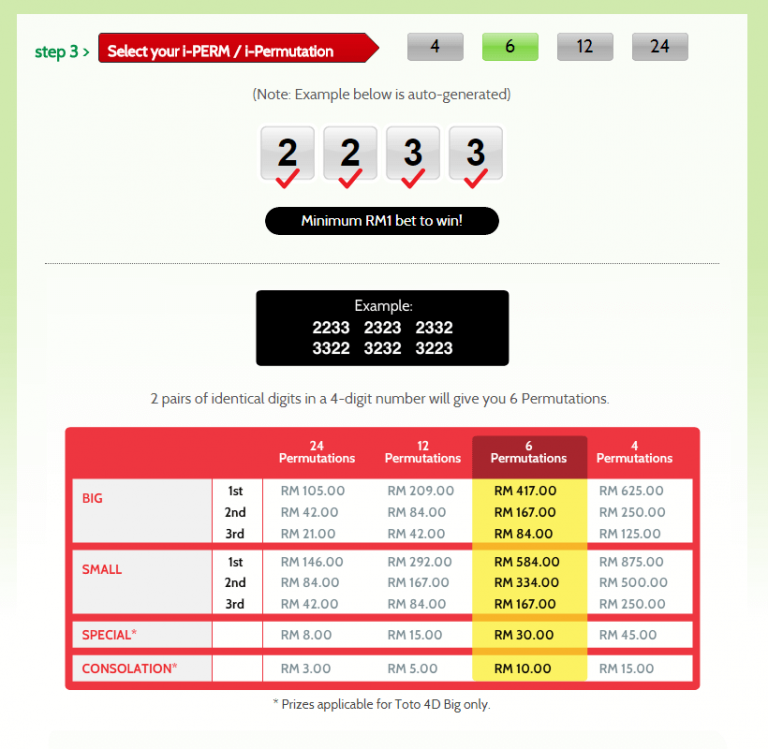Below is an example of a 12-Permutation number which costs RM1.00.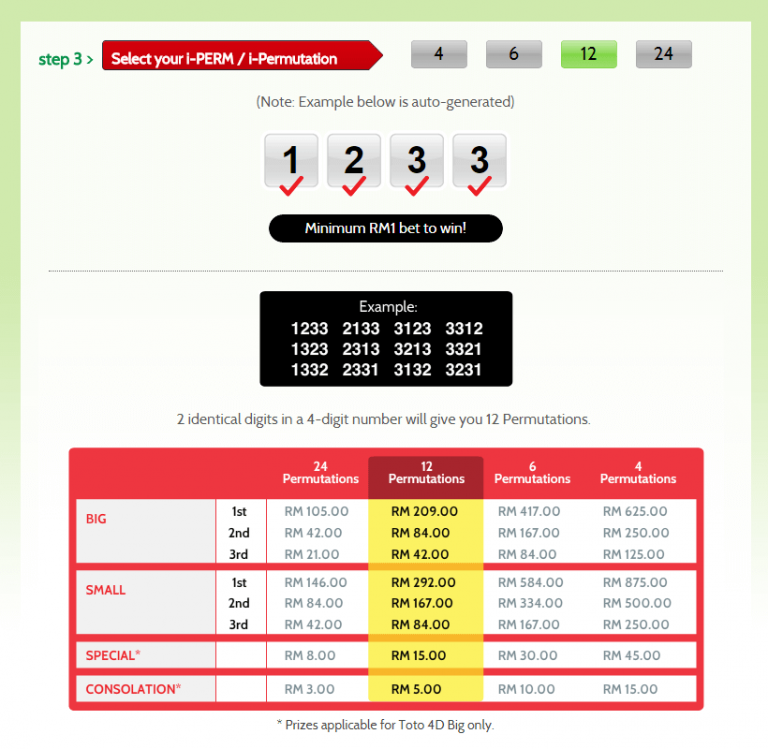Below is an example of a 24-Permutation number which costs RM1.00.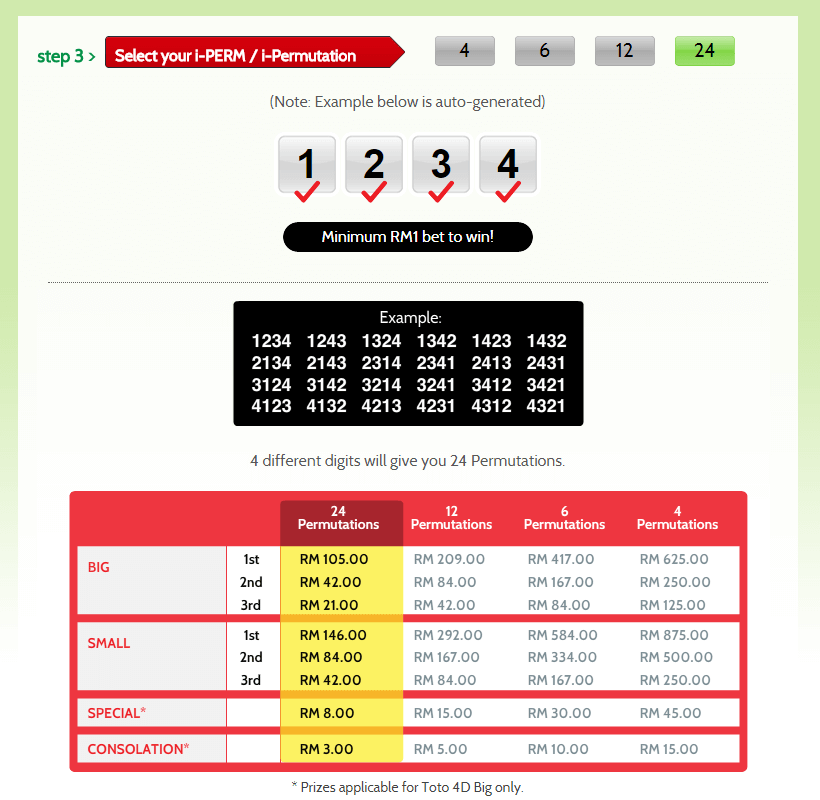#### 4D Roll

Below is an example of a 4D Roll 1 number that costs RM10.00 minimum to play.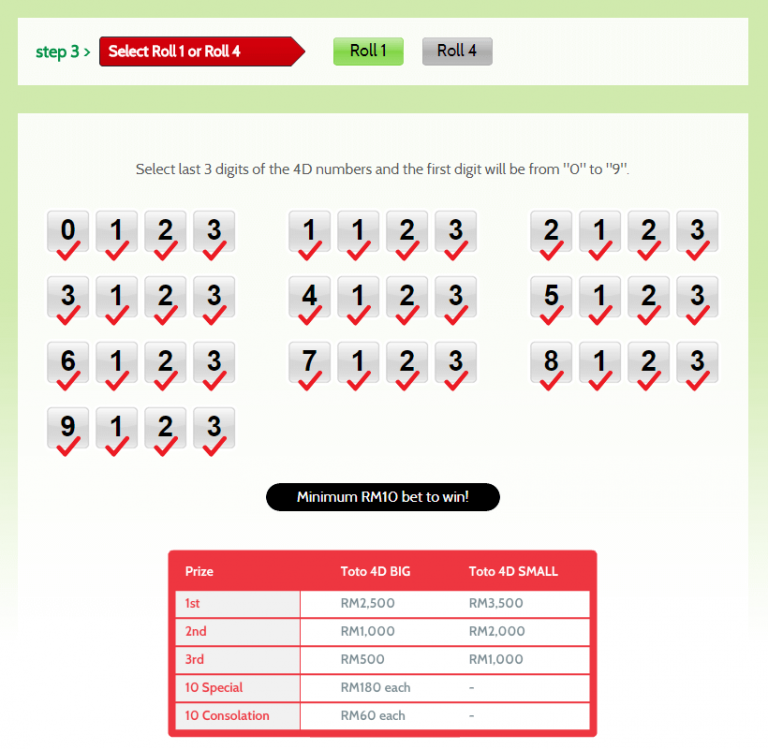Below is an example of a 4D Roll 4 number that costs RM10.00 minimum to play.